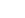# Problem: What is the standard electrode potential for a voltaic cell constructed in the appropriate way from these two half-cells?  Standard Reduction Potentials                   E°      Cr 3+ (aq) + 3e– → Cr(s)                        – 0.74 V Co 2+ (aq) + 2e– → Co(s)                     – 0.28 V   A) – 1.02 V B) 0.46 V C) 0.64 V D) 1.02 V

###### FREE Expert Solution
79% (170 ratings)
###### Problem Details

What is the standard electrode potential for a voltaic cell constructed in the appropriate way from these two half-cells?

Standard Reduction Potentials                   E°

Cr 3+ (aq) + 3e → Cr(s)                        – 0.74 V

Co 2+ (aq) + 2e– → Co(s)                     – 0.28 V

A) – 1.02 V

B) 0.46 V

C) 0.64 V

D) 1.02 VWhat scientific concept do you need to know in order to solve this problem?

Our tutors have indicated that to solve this problem you will need to apply the Cell Potential concept. If you need more Cell Potential practice, you can also practice Cell Potential practice problems.

What is the difficulty of this problem?

Our tutors rated the difficulty ofWhat is the standard electrode potential for a voltaic cell ...as low difficulty.

How long does this problem take to solve?

Our expert Chemistry tutor, Jules took 1 minute and 48 seconds to solve this problem. You can follow their steps in the video explanation above.

What professor is this problem relevant for?

Based on our data, we think this problem is relevant for Professor Schvaneveldt's class at CLEMSON.